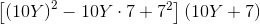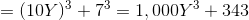# PSAT Math : How to multiply polynomials

## Example Questions

### Example Question #1 : How To Multiply Polynomials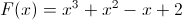and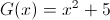What is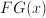?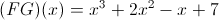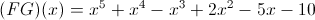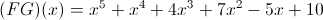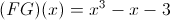Explanation: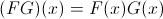so we multiply the two function to get the answer.  We use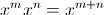### Example Question #7 : Multiplying And Dividing Polynomials

Multiply: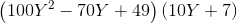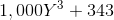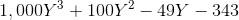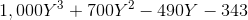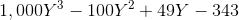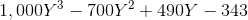Explanation: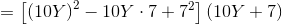This product fits the sum of cubes pattern, where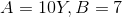: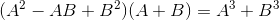So